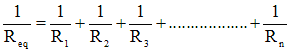Resistors in series|parallel combinations

(10)Combination of Resistors

• We have earlier studied that sevral capacitors can be connected in series or parallel combination to form a network. In same way sevral resistor may be combined to form a network.
• Just like capacitors resistors can be grouped in series and parallel.
• Equivalent resistance of the combination of any number of resistors is a single resistance which draw same current as the combination of different resistances draw when the same potential difference is applied across it.

(A) Resistors in Series
• Resisors are said to be connected in series combinaton. If same current flows through each resistor when same potential difference is applied across the combination.
• Consider the figure given below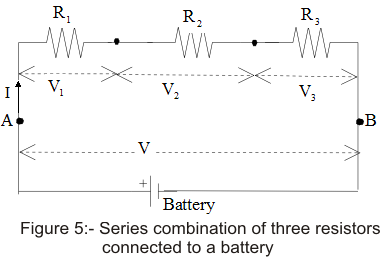• In figure given above three resistors if resistance R1, R2 and R3 are connected ibn series combination.
• If battery is connected across the series combination so as to mintain potential difference V between points A and B, the current I would pass through each resistor.
• If V1, V2 andV3 is the potential difference across each resistor R1, R2 and R3 respectively, then according to Ohm's Law,
V1=IR1
V2=IR2
V3=IR3
Since in series combination current remains same but potential is divieded so,
V=V1+V2+V3
or, V=I(R1+R2+R3)
If Reqis the resistance equivalent to the series combination of R1, R2 and R3 then ,
V=IReq
where, Req=R1+R2+R3
• Thus when the resistors are connected in series, equivalent resistance of the series combination is equal to the sum of individual resistances.
• Value of esistance of the series combination is always greater then the value of largest individual resisnces.
• For n numbers of resistors connected in series equivalent resistance would be
Req=R1+R2+R3+...........................+Rn

(B) Resistors in parallel
• Resistors are said to be connected in parallel combination if potential difference across each resistors is same.
• Thus , in parallel combination of resistors potential remains the same but current is divided.
• Consider the figure given below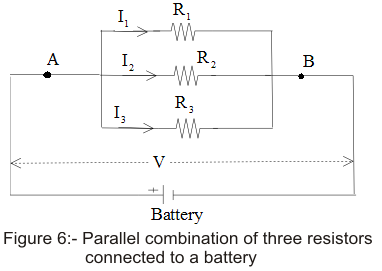• Battery B is connected across parallel combination of resistors so as to maintain potential difference V across each resistors.Then total current in the circuit would be
I=I1+I2+I3                           (16)
• Since potential difference across each resistors is V. Therefore, on applying Ohm's Law
V=I1R1=I2R2=I3R3
or,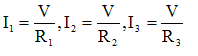From equation (16)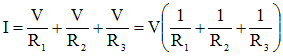• If R is the equivalent resistance of parallel combination of three resistors heaving resistances R1, R2 and R3 then from Ohm's Law
V=IReq
or,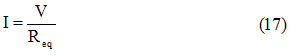Comparing equation (16) and (17) we get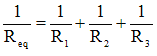• For resistors connected in parallel combination reciprocal of equivalent resistance is equal to the sum of reciprocal of individual resistances.
• Value of equivalent resistances for capacitors connected in parallel combination is always less then the value of the smallest resistance in circuit.
• If there are n number of resistances connected in parallel combination, then quivalent resistance would be reciprocal of